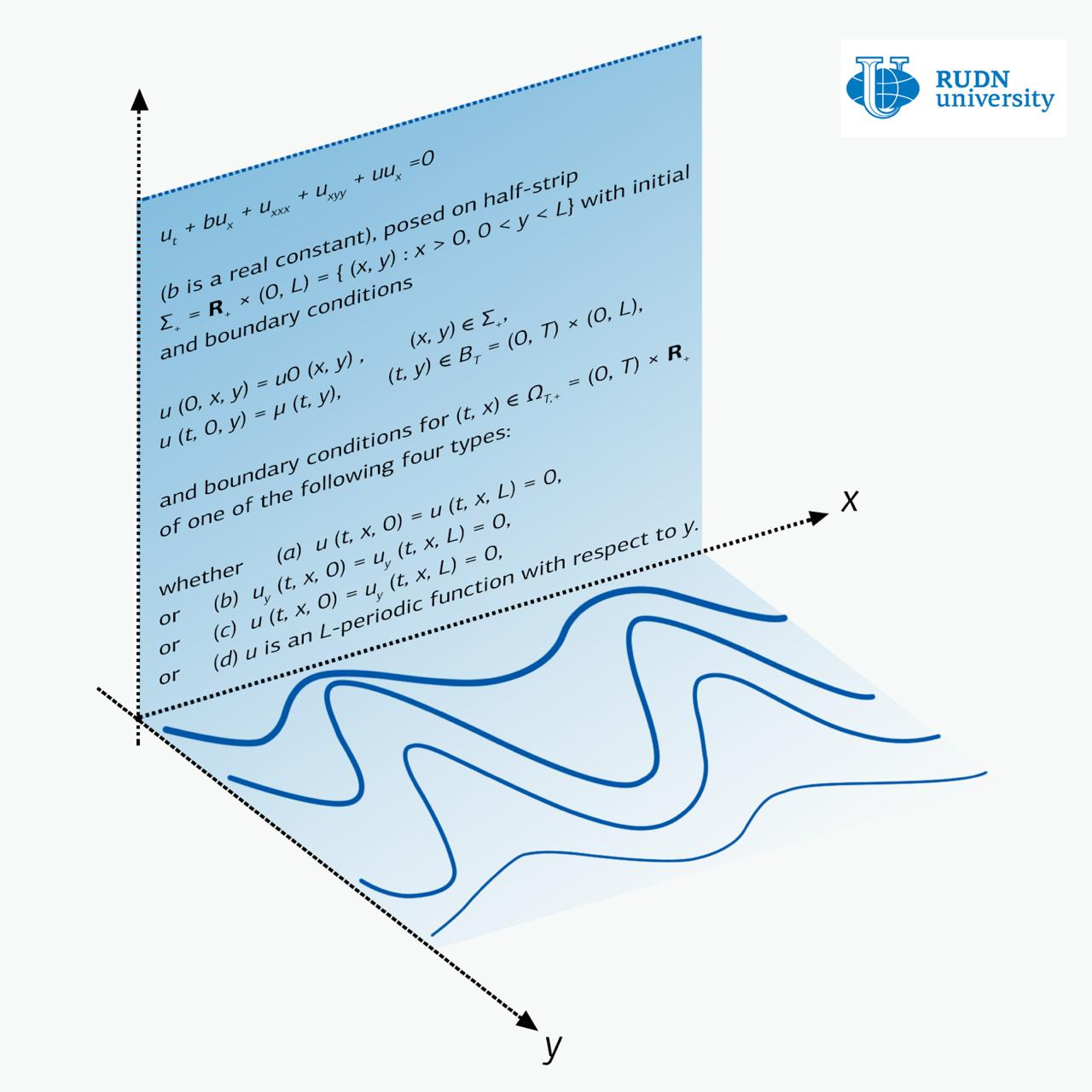RUDN University mathematician first described the movement in a flat strip of plasma

# RUDN University mathematician first described the movement in a flat strip of plasma

RUDN University mathematician for the first time proved the theorem of existence and uniqueness of solutions of the Zakharov-Kuznetsov equation in a strip. Such theorems are very rare for partial differential equations. The new results can be applied, for instance, in astrophysics, in describing the propagation of plane waves in plasma. The article is published in the journal Nonlinear Analysis: Real World Applications.

The Zakharov-Kuznetsov equation is a one-function equation of two variables x and y. For physics, x is the direction of wave propagation, and the deformation of the medium occurs along the perpendicular direction y. For example, an oscillation of the guitar string looks like the wave runs down the string, while the oscillations occur perpendicular direction relative to the run of the wave.

There are a large number of results that describe solutions of the Zakharov-Kuznetsov equations in the case when there are no constraints on y. But the question of wave propagation in the strip — when y is limited — was almost not studied until recently. And this is although such a statement of the problem has a physical meaning, and therefore potential applications.

RUDN University mathematicians dealt with the Zakharov-Kuznetsov equation in the strip. They examined three main cases — when there are no oscillations on the boundary of the strip, when there is no current on the same boundary and when the boundary conditions are periodic in structure. The latter case corresponds to the propagation of waves in a medium whose structure is periodic in x.

In all these cases mathematicians managed to prove theorems of existence and uniqueness of solutions. For systems of partial differential equations, which include the Zakharov-Kuznetsov equation, such equations are very rare.

These results are the first for solutions of the equation with initial conditions in the strip. Flat plasma flows with boundary conditions, which were considered by RUDN University scientists, can occur in physics and astrophysics.

The Zakharov-Kuznetsov equations belong to a wider category of equations known as the Korteweg-de Vries equations. In the study of this category of equations for the first time, it was possible to describe solitons — waves whose shape does not change during movement. Physicists consider solitons as a tool for modern optical data transmission systems. The study of solitons, which can arise in the Zakharov-Kuznetsov equations, is one of the options for the development of the work done by RUDN University mathematicians.News
All news
Science
27 Apr
Vocabulary Size Affects One’s Ability to Differentiate Between Vowels in a Foreign Language, Say Linguists from RUDN University

A team of linguists from RUDN University established that a person’s ability to accurately differentiate between vowel sounds of a foreign language correlates with the size of their vocabulary in said language.

Science
22 Apr
A Bifidobacterial Protein Capable of Reducing Inflammation in Patients with COVID-19 and Other Diseases Was Discovered by a RUDN University Geneticist

A geneticist from RUDN University studied the effect of Bifidobacterium (intestinal bacteria) on the inflammatory process and discovered that their surface protein is capable of stopping excessive or uncontrollable inflammation, like the one observed in COVID-19 patients. A fragment of this protein can be used as an anti-inflammatory medication when treating coronavirus and other diseases.

Science
13 Apr
RUDN University mathematician suggested new approach to cooperative game

A mathematician from RUDN University developed a matrix representation of set functions. This approach is vivid and easy to check, and it makes the calculations easier. Among other things, the new development can be applied to cooperative game theory.